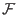Next: Shor's Algorithm Up: The Quantum Computer Previous: Probability Interpretation   Contents

## Quantum Parallelism

Quantum parallelism arises from the ability of a quantum memory register to exist in a superposition of base states. Each component of this superposition may be thought of as a single argument to a function. A function performed once on the register in a superposition of states is performed on each of the components of the superposition. Since the number of possible states is 2n where n is the number of qubits in the quantum register, you can perform in one operation on a quantum computer what would take an exponential number of operations on a classical computer. This is fantastic, but the more superposed states that exist in you register, the smaller the probability that you will measure any particular one will become.

As an example suppose that you are using a quantum computer to calculate the function(x) = 2*x mod 7, where x is the superposition of integers between 0 and 7 inclusive. You could prepare a quantum register that was in a equally weighted superposition of the states 0-7. Then you could perform the 2*x mod 7 operation once, and the register would contain the equally weighted superposition of 1,2,4,6,1,3,5,0 states, these being the outputs of the function 2*x mod 7 for inputs 0 - 7. When measuring the quantum register you would have a 2/8 chance of measuring 1, and a 1/8 chance of measuring any of the other outputs. It would seem that this sort of parallelism is not useful, as the more we benefit from parallelism the less likely we are to measure a value of a function for a particular input. Some clever algorithms have been devised, most notably by Peter Shor which succeed in using quantum parallelism on a function where there is interest in some property of all the inputs, not just a particular one.

This kind of parallelism is very appealing for simulation on a parallel computer. A n bit quantum register contains a superposition of each of its 2n possible base states, and we represent this by an array of 2n complex numbers which are probabilities of measuring the quantum register to be the corresponding base state. To perform an operation on the quantum register, we simply modify each of the 2n array locations. By splitting the calculations of how to change the probability values of the array locations into even ranges via process elements, we get nearly linear speedup.Next: Shor's Algorithm Up: The Quantum Computer Previous: Probability Interpretation   Contents
Matthew Hayward - Quantum Computing, Shor's Algorithm, and Parallelism GitHub Repository